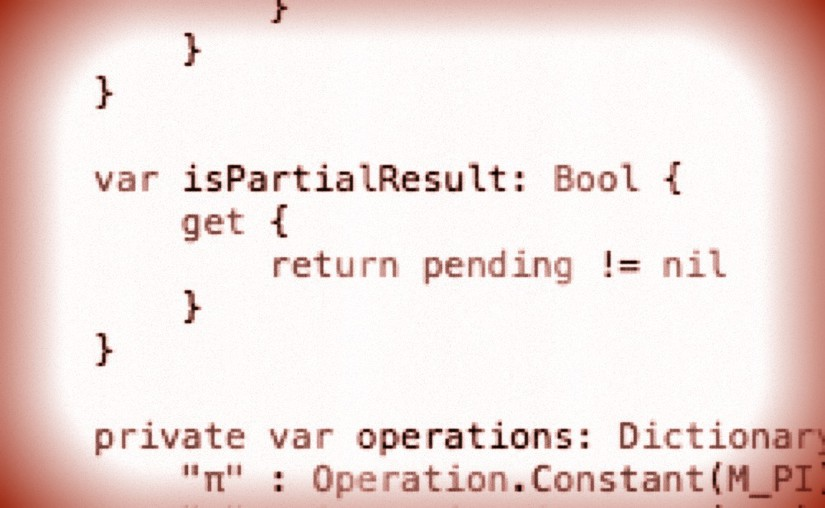# cs193p – Assignment #1 Task #6Please note, this blog entry is from a previous course. You might want to check out the current one.

Add a Bool property to your CalculatorBrain called isPartialResult which returns whether there is a binary operation pending (if so, return true, if not, false).

This a a short one, just add a computed property depending on the private pending property:

```var isPartialResult: Bool {
get {
return pending != nil
}
}
```

… and adjust the unit tests:

```func testDescription() {
// a. touching 7 + would show “7 + ...” (with 7 still in the display)
...
XCTAssertTrue(brain.isPartialResult)

// b. 7 + 9 would show “7 + ...” (9 in the display)
...
XCTAssertTrue(brain.isPartialResult)

// c. 7 + 9 = would show “7 + 9 =” (16 in the display)
...
XCTAssertFalse(brain.isPartialResult)

// d. 7 + 9 = √ would show “√(7 + 9) =” (4 in the display)
...
XCTAssertFalse(brain.isPartialResult)

// e. 7 + 9 √ would show “7 + √(9) ...” (3 in the display)
...
XCTAssertTrue(brain.isPartialResult)

// f. 7 + 9 √ = would show “7 + √(9) =“ (10 in the display)
...
XCTAssertFalse(brain.isPartialResult)

// g. 7 + 9 = + 6 + 3 = would show “7 + 9 + 6 + 3 =” (25 in the display)
...
XCTAssertFalse(brain.isPartialResult)

// h. 7 + 9 = √ 6 + 3 = would show “6 + 3 =” (9 in the display)
...
XCTAssertFalse(brain.isPartialResult)

// i. 5 + 6 = 7 3 would show “5 + 6 =” (73 in the display)
...
XCTAssertFalse(brain.isPartialResult)

// j. 7 + = would show “7 + 7 =” (14 in the display)
...
XCTAssertFalse(brain.isPartialResult)

// k. 4 × π = would show “4 × π =“ (12.5663706143592 in the display)
...
XCTAssertFalse(brain.isPartialResult)

// m. 4 + 5 × 3 = could also show “(4 + 5) × 3 =” if you prefer (27 in the display)
...
XCTAssertFalse(brain.isPartialResult)
}
```

The complete code for task #6 is available on GitHub.